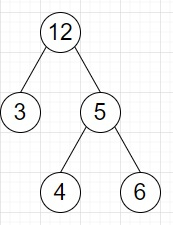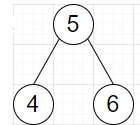# Program to find largest binary search subtree from a given tree in Python

Suppose we have a binary tree, we have to find the largest subtree (with maximum number of nodes) as binary search tree.

So, if the input is likethen the output will beTo solve this, we will follow these steps −

• max_size := 
• max_node := [null]
• Define a function traverse() . This will take node
• if node is null, then
• return null
• left := traverse(left of node)
• right := traverse(right of node)
• lst := left + [value of node] + right
• if lst is sorted, then
• if max_size < size of lst, then
• max_size := size of lst
• max_node := node
• return lst
• traverse(root)
• From the main method return max_node

## Example (Python)

Let us see the following implementation to get better understanding −

class TreeNode:
def __init__(self, data, left = None, right = None):
self.val = data
self.left = left
self.right = right
def print_tree(root):
if root is not None:
print_tree(root.left)
print(root.val, end = ', ')
print_tree(root.right)
class Solution:
def solve(self, root):
max_size = 
max_node = [None]
def traverse(node):
if not node:
return []
left = traverse(node.left)
right = traverse(node.right)
lst = left + [node.val] + right
if sorted(lst) == lst:
if max_size < len(lst):
max_size = len(lst)
max_node = node
return lst

traverse(root)
return max_node
ob = Solution()
root = TreeNode(12)
root.left = TreeNode(3)
root.right = TreeNode(5)
root.right.left = TreeNode(4)
root.right.right = TreeNode(6)
print_tree(ob.solve(root))

## Input

root = TreeNode(12)
root.left = TreeNode(3)
root.right = TreeNode(5)
root.right.left = TreeNode(4)
root.right.right = TreeNode(6)

## Output

4, 5, 6,